﻿ Numerical Simulation of Natural Convection in a Vertical Conical Cylinder Partially Annular Space

### Numerical Simulation of Natural Convection in a Vertical Conical Cylinder Partially Annular Space

Belkacem OULD SAID, Noureddine RETIEL, El Hadi BOUGUERRAOPEN ACCESSPEER-REVIEWED

## Numerical Simulation of Natural Convection in a Vertical Conical Cylinder Partially Annular Space

Belkacem OULD SAID1, Noureddine RETIEL1,, El Hadi BOUGUERRA2

1Laboratory Numerical and Experimental Modeling of Mechanical phenomena, Mechanical Engineering Department, Abdelhamid Ibn Badis University, Mostaganem, Algeria

2Mechanical Engineering Department, Saad Dahlab University, Blida, Algeria.

### Abstract

The present paper is dedicated to the numerical simulation of thermal convection in a two dimensional vertical conical cylinder partially annular space. The governing equations of mass, momentum and energy are solved using the CFD FLUENT code. The results of streamlines and the isotherms of the fluid are discussed for different annuli with various boundary conditions and Rayleigh numbers. Emphasis was put on the height of the inner vertical cylinder influence on the flow and the temperature distribution. More, the effects on the heat transfer are analyzed for different values of the fluid’s physical parameters in the annulus geometry. The heat transfer on the hot wall of the annulus is also computed in order to make comparisons the cylinder annulus for boundary conditions and several Rayleigh numbers. The obtained results in terms of Nusselt number has been found between the present previsions and available data from the published literature data.

### At a glance: Figures

123
Prev Next

• SAID, Belkacem OULD, Noureddine RETIEL, and El Hadi BOUGUERRA. "Numerical Simulation of Natural Convection in a Vertical Conical Cylinder Partially Annular Space." American Journal of Energy Research 2.2 (2014): 24-29.
• SAID, B. O. , RETIEL, N. , & BOUGUERRA, E. H. (2014). Numerical Simulation of Natural Convection in a Vertical Conical Cylinder Partially Annular Space. American Journal of Energy Research, 2(2), 24-29.
• SAID, Belkacem OULD, Noureddine RETIEL, and El Hadi BOUGUERRA. "Numerical Simulation of Natural Convection in a Vertical Conical Cylinder Partially Annular Space." American Journal of Energy Research 2, no. 2 (2014): 24-29.

 Import into BibTeX Import into EndNote Import into RefMan Import into RefWorks

### 1. Introduction

The natural convection in an enclosure is a large research topic due to its wide variety of engineering applications involving energy conversion, storage and transmission systems. Annular space cavities are used in solar collectors  and the nuclear reactor design. A comprehensive review of natural convection in various cavity shapes has been documented in the literature. Among the very first investigations, Wang  has analyzed numerically the heat transfer problem by natural convection in a rectangular enclosure filled by micropolar fluid, and particularity studied the influence of the conductive vertical divider. The case of square and cubic cavities was reported by [3, 4]. Other investigations  have been conducted used CFD simulations to study the effect of the physical and geometrical parameters in two-dimensional vertical enclosure. The complex shapes such that inclined cavities with wavy walls by Aounallah , and trapezoidal cavities by Varol . Natural convection and fluid flow was studied for triangular enclosures mostly with boundary conditions, see [8, 9]. Varol  have carried investigations of heat transfer by protruding isothermal heater within a triangular enclosure. The numerical study of the phenomenon of natural convection flow in a vertical concentric annular with isothermal inner and outer vertical walls have been conducted by various authors [11, 12, 17, 18]. Several problems that have been extensively studied due to their several practical applications have received much attention. Hammad  analyzed the effect of tilted angle and diameter ratio on natural convection heat transfer in the case of horizontal cylindrical annulus. Investigations on the transition effect and turbulence flows on natural convection along a horizontal annular cavity with local and mean Nusselt numbers were presented by  and recently by . Few researches has been done to conical shape, we include a numerical study on heat transfer by natural convection and radiation in conical annular porous cylinder and presented by Salman, N.J.A. & al. . These studies were restricted to conduction heat transfer only. The present paper covers the laminar natural convection in a vertical conical cylinder partially annular space. On the other hand, the direct numerical simulation (DNS) approach necessary for a good resolution of the heat transfer problems requires computational resources beyond actual computing capacities dedicated for real industrial problems. We will be concerned also with the effect of the Rayleigh number, radius ratio and height ratio of annulus as well as the cavity geometry on the heat transfer.

### 2. Mathematical formulation

2.1. Physical Domain

In the present problem, the geometry is schematized for the flow produced by natural convection in a vertical conical cylinder partial annulus where the annulus is filled with air. The analysis domain is delimited by two concentric cone and a cylinder with isothermal walls of the inner and outer axial height h and H, respectively. The top and bottom walls of the outer cone are considered adiabatic as shown in figure 1. The bottom radius of inner cylinder and outer cone are rRi Rand rRoR and the inner and outer wall temperature are Ti and To where TRi>TRoR. The buoyancy induced flow is assumed to be laminar, incompressible with constant fluid properties except the density variation. The Boussinesq approximation is used to calculate the density variation with the temperature. Then, the mathematical model used is based on the hypothesis of a two-dimensional (axisymetric) flow.

2.2. Governing Equations

The problem description and assumptions on the fluid properties are given as governing differential equations (vector form) and can be written as:

Continuity,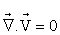(1)

Momentum,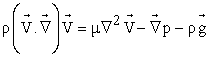(2)

Energy,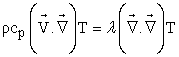(3)

Those governing equations can be written in dimensionless cylindrical coordinates form.

Continuity,(4)

R momentum,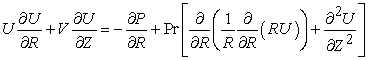(5)

And Z momentum with the Boussinesq approximation for the buoyancy term,(6)

Energy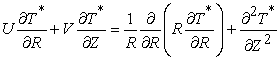(7)

Where the dimensionless parameters are defined as follows: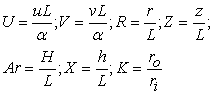(8)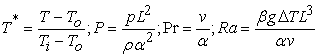(9)
2.3. Boundary Conditions

The corresponding dimensionless boundary conditions for the radial and vertical velocity are equals to zero at all walls. The temperature boundary conditions are as follows.

At 0≤ Z≤ H/L and RRoR≤R≤RRoR-(H/L)cotgδ: U=V=0 and T*=0 for the isothermal Cold tilted wall

At 0≤ Z≤ h/L and R=RRiR: U=V=0 and T*=1 for the isothermal hot vertical wall

At Z= h/L and 0≤R≤RRiR: U=V=0 and T*=1 for the isothermal hot horizontal wall

At Z=H/L and Z =0: U= V= 0 and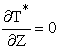for the adiabatic walls

### 3. Numerical Method

To solve the governing equations, the finite volume method was used. The FLUENT CFD code with first order formulation was used with the segregated implicit scheme. The conservation governing equations was solved independently by the segregated solver. The first order upwind differencing scheme is used for the equations of momentum and energy. The discretization scheme used for pressure was body force weighted to take account for the density variations. The pressure–velocity coupling is ensured using the SIMPLE algorithm. Gambit was used to create and mesh the geometrical model with a simple quadrilateral cell. If necessary, a very fine spacing near the walls in the conical cylinder partially annular grid for correctly resolving the steep gradients in the thin buoyancy has driven boundary layer. All the variables were calculated right up to the walls without using any wall function. On the wall boundary conditions, the radial and vertical velocity values were set to zero. The dimensionless temperatures of the active walls are set to 0 and 1 respectively. While meshing the domain, care is taken so that the mesh size does not influence the solution. The residuals of continuity, momentum and energy equations are required to be lower 10P-7P in order to achieve totally the convergence of the solution. The relaxation parameters have been adapted for every simulation in order to speed up convergence.

### 4. Results and Discussion

4.1 Nusselt Numbers and Validation

The heat transmitted by the inner cylinder of the annulus is quantified by the local and mean Nusselt numbers. The local Nusselt number for the annulus inner cylinder is obtained from temperature gradients by the following relations:(10)

Whereas the mean Nusselt number is defined by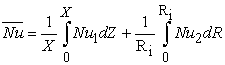(11)

It's necessary to ensure a precision of the present numerical model and the FLUENT CFD code. The heat transfer data computed for differentially heated conical annular, with different parameters that correspond to the cone angle δ=90, corresponding to a cylinder, aspect ratio Ar=H/L, radius ratio K=rRoR/rRiR, height ratio h=X/L and Rayleigh number 10P4P≤ Ra ≤10P5P. The present problem is to numerically investigate the natural convection flow in a vertical cylinder annular space and the average Nusselt number variation is compared with references from the literature data. Table 1 shows the comparison between the current results and those of [17, 18]. It is clearly demonstrated that for several values of Rayleigh number there is a satisfactory agreement between the present results and those obtained by these authors.

#### Table 1. Values of the overall Nusselt number at the isothermal walls for annulus aspect ratio Ar=10 and K=2, δ=90°Download asPowerPoint Slide

Veiw figureView current table in a new window

4.2. Effect of Rayleigh Number

The Effects of Rayleigh number on streamlines (on the left) and isotherms (on the right) can be seen in figure 2 for K= 10, X=0.5 and δ=60P. Visualizations are given from Ra=10P3 Pto Ra=10P6P. As it can be seen from these figures, for low values of the Rayleigh number (Ra=10P3P), the unicellular structure is formed, for all values of the Rayleigh numbers considered, which the direction is in rotation clockwise. At lower Rayleigh number Ra≤ 10P4P such that Figure 2 a-b the isotherms are almost vertical at the active wall for most of the annulus. Illustrating the mechanism of heat transfer flow where conduction dominates. For higher values of the Rayleigh number (10P5P≤ Ra≤ 10P6P), an upward flow accelerated at the hot wall and descendant slow along inclined height of the cold wall as shown in figure 2 c-d, due to the increase of the mode of convection heat transfer, the steep thermal gradient were formed near the left wall and weak at the right top of the annulus for 1≤ R≤ 2. It's clearly shown in the velocity and the temperature profiles Figure 3 and Figure 4 respectively at Z=0.75.

The velocity profiles are presented in Figure 3 for different Rayleigh numbers ranging from Ra=10P3P to Ra=10P6P and several annulus radius ratio K= 2, 5, 10. Profiles are plotted along the horizontal direction. Other geometric parameters were chosen as X=0.5 and δ=60PP. But, when increasing annulus radius ratio, the fluid velocity is begins to accelerate from the base and forms the primary flow in the vertical direction to up at the axis symmetry of the annulus, and achieve the maximum value for K=10 at (0 ≤ R ≤ 0.44). As the R=1.57 near the cold wall, the particules of fluid go down slowly.

The isotherms (on the right) and streamlines (on the left) are depicted variations for annulus radius ratio K As can be seen from Figure 5. The figure obtained for different radius ratio values i.e. K=2, 5 & 10 and δ=60P, corresponding to Ra = 10P5P. The form of the isotherms show that increasing the annulus radius ratio provoked to the isotherms crowding at the bottom left of the annulus. The temperature gradient in the cold wall can also be seen from the isothermal continuous decrease as the increase in the annulus radius ratio. We remark the temperature gradient very important at the hot wall Figure 5a indicates that the fluid heated up better when the radius ratio is low, which is not the case at higher radius ratio Figure 5c. The fluid flow center moves to right of annulus when radius ratio increases.

The increase in Nusselt number for various annulus radius ratio K=2, 5 & 10 and annulus height ratio X=0.5 & δ=60 and their linear variation with Rayleigh number on a logarithmic scale are depicted in figure 6. As expected, the average Nusselt number increases as the Rayleigh number is increased by the growing contribution of natural convection. The heat transfer rate increases also with decrease in the annulus radius ratio.

The Nusselt number evolution with annulus radius ratio K=2, 5 and 10 for different Rayleigh number and X=0.5 and δ=60 are plotted in figure 7. The average heat transfer rate for different annulus radius ratio. It's found that the Nusselt number increases as the annulus radius ratio decreases, which means that the heat transfer rate decreases as the hot wall surface decreases (increases in annulus radius ratio K).

4.4. Effect of the Height Ratio

The isotherms (on the right) and streamlines (on the left) with different annulus height ratio X are shown in figure 8. This figure corresponds to the several values Ra=10P5P, K=2 and δ=60P. It can be clearly noted from this figure that the isotherms move to the hot wall with a annulus height ratio increase. This denotes that increasing of the annulus height ratio leads to increasing the heat transfer from the hot wall of annulus. The temperature gradient at the hot wall can also be seen from the isothermal continuous decrease as the decrease in the annulus height ratio. The fluid flow is clearly visualized in the streamlines. The complete cycle were formed through the fluid circulation directed upward toward the hot wall and falls then to the cold wall of the annulus showing that the fluid flow concentrates of the circular cell at the all of annular space of small annulus height ratio decreases. But it moves in half of the annular space (1≤ R ≤2), when the annulus height ratio increases and the cell orientation becomes parallel to the vertical inner cylinder.

The Nusselt number variation with aspect ratio X for several Rayleigh number are shown in figure 9 corresponding to K = 2 and δ=60. As expected, the heat transfer rate is greater at the Rayleigh number is important. It can be inferred from the figure that the growth rate effect is higher than the line for Ra ≤105 is stronger at higher Rayleigh number values and the line corresponding to Ra=106. The annulus height ratio does not influence the heat transfer rate when Ra ≤10P5P, because the fluid move is very slow, and therefore the conductive regime prevails over convection regime, which explains the almost constant value of the Nusselt number.

Figure 9. Nusselt number variation with annulus height ratio for different Rayleigh number at K=2, δ=60

### 5. Conclusion

In this paper, the numerical study of natural convection in a two dimensional vertical conical cylinder partially annular space for steady-state regime with differentially heated walls has been analyzed. The effect of main parameters as Rayleigh number, radius ratio and Height ratio of annulus are also investigated. We can concisely summaries the major results as following:

We have highlighted the effect of Rayleigh number on behavior the flow structures and the temperature field in steady regime. Two flow regimes were observed for lower Rayleigh number values and a dominance of conduction heat transfer. At higher values of Rayleigh number, heat transfer rate is increased and are rather dominated by convection mode.

The annulus radius ratio is one of the more important parameters on flow and temperature fields and heat transfer. Thus, the average heat transfer rate is favourable for a low annulus radius ratio.

The heat transfer rate increases with Rayleigh number increase. The heat transfer rate decreasing as the annulus height ratio decrease.

For high values of the annulus height ratio, the cell orientation becomes parallel to the vertical inner cylinder i.e. the fluid motion is accelerated fully in the 1≤R≤2 and they is almost zero in the other side 0≤R≤1 at above the inner cylinder. But when height ratio decreases the fluid motion is accelerated increasingly in the zone 0≤R≤1 (the circular cell of the fluid motion is concentrated at the all in this zone).

### Nomenclature

Ar=H/L: Aspect ratio

g: Acceleration of gravity (m/s2)

h: Height of the inner conical (m)

H: Height of the outer conical (m)

L: Annulus gab width=(ro-ri) (m)

Nu: Nusselt number

P: Static pressure (N/m2)

P*: Dimensionless pressure

Pr: Prandtl number

Ra: Rayleigh number

ri: Inner radii at bottom (m)

ro: Outer radii at bottom (m)

Ri: Dimensionless Inner radii at bottom

Ro: Dimensionless Outer radii at bottom

T: Local temperature (°K): Dimensionless temperature

Ti,, To: Temperatures at inner and outer wall (°K)

ΔT: Temperature difference (°K)

u: Velocity in the radial direction (m/s)

v: Velocity in the axial direction (m/s)

U: Dimensionless velocity in the radial direction

V: Dimensionless velocity in the axial direction

X=h/L: Height ratio

z: Axial coordinate

Z: Dimensionless axial coordinate

Greek symbols

α: Thermal diffusivity (m2/s)

β: Coefficient of thermal expansion (1/°K)

ρ: Fluid density (kg/m3)

ν: Kinematic viscosity (m2/s)

μ: Dynamic viscosity (kg/ms)

λ: Fluid thermal conductivity (w /m°K)

Subscripts

  Sridhar, A. and Reddy, K.S., Transient analysis of modified cuboid solar integrated-collector-storage system, Applied Thermal Engineering., Vol. 27, 330-346, 2007.In article CrossRef  Wang, S.G. and Li, T. Y. and Hsu, P.T. and Kaohsiung and Taiwan, Natural convection of micropolar fluid in a partially divided enclosure, Acta Mechanica., Vol. 136, 41-53, 1999.In article CrossRef  He, 5TY. L. and Yang, 5TW. W5T. And Tao, W. Q., Three-Dimensional Numerical Study of Natural Convective Heat Transfer of Liquid in a Cubic Enclosure, Numerical Heat Transfer Part A Applications., Vol. 47, 917-934, 2005.In article CrossRef  Dixit, H.N., Babu,V., Simulation of high Rayleigh number natural convection in a square cavity using the lattice Boltzmann method, International Journal of Heat and Mass Transfer. Vol. 49, 727-739, 2006.In article CrossRef  Ganguli, A.A. and Pandit, A. B. and Joshi, J. B., CFD simulation of heat transfer in a two-dimensional vertical enclosure, Chemical Engineering Research and Design, Vol. 87, 711-727, 2009.In article CrossRef  Aounallah, M. and Addad, Y. and Benhamadouche, S. and Imine, O. and Adjlout, L. and Laurence, D., Numerical investigation of turbulent natural convection in an inclined square cavity with a hot wavy wall, International Journal of Heat and Mass Transfer, Vol. 50, 1683-1693, 2007.In article CrossRef  Varol, Y. and Oztop, H. F. and Pop, I., 5TNatural convection in right-angle porous trapezoidal enclosure partially cooled from inclined wall, International Communications in Heat and Mass Transfer, Vol. 36, 6-15, 2009.In article CrossRef  Fuad Kent, E. and Asmaz, E. and Ozerbay, S., Laminar natural convection in right triangular enclosures, Heat and Mass Transfer, Vol. 44, 187-200, 2007.In article CrossRef  Hajri, I. and Omri, A. and Ben Nasrallah, S., A numerical model for the simulation of double-diffusive natural convection in a triangular cavity using equal order and control volume based on the finite element method, Desalination, Vol. 206,5T 579-588, 2007.In article  Varol, Y. and Oztop, H.F. and Yilmaz, T., 5Natural convection in triangular enclosures with protruding isothermal heater, International Journal of Heat and Mass Transfer, Vol. 505T, 2451-2462, 2007.In article CrossRef  Hadjadj, A. and Maamir, S. and Zeghmati, B., A new study of laminar natural convection in two concentric vertical cylinders, Heat and Mass Transfer, Vol. 35, 113-121, 1999.In article CrossRef  Venkata Reddy, P. and Narasimhamn, G. S. V. L., Natural convection in a vertical annulus driven by a central heat generating rod, International Journal of Heat and Mass Transfer, Vol. 51, 5024-5032, 2008.In article CrossRef  Hamad, F.A. and Khan, M.K., Natural convection heat transfer in horizontal and inclined annuli of different diameter ratios, Energy Conversion and Management, Vol. 39, 797-807, 1998.In article CrossRef  Padilla, E.L.M. and Silveira-Neto, A., Large-eddy simulation of transition to turbulence in natural convection in a horizontal annular cavity, International Journal of Heat and Mass Transfer, Vol. 51, 3656-3668, 2008.In article CrossRef  Habibi Matin, M. and Pop, I., 2013, Natural convection flow and heat transfer in an eccentric annulus filled by Copper nanofluid, International Journal of Heat and Mass Transfer, Vol.5T615T, 353-364, 2008.In article  Salman, N.J.A. and Irfan, A.B. and Zainal, Z.A. and Khaleed, H.M.T. and Jeevan, K., Heat transfer in a conical cylinder with porous medium, International Journal of Heat and Mass Transfer, Vol. 52, pp, 3070-3078., 2009.In article  G. de Vahl Davis5T9T, 9T5TR. W. Thomas5T, 11TNatural Convection between Concentric Vertical Cylinders”, 11T5TPhysics of Fluids5T12T, Vol. 12, 198-207 1969In article  Kumar, R. and Kalam, M.A., Laminar Thermal Convection between Vertical Coaxial Isothermal Cylinders”. International Journal of Heat and Mass Transfer, Vol. 34, 513-524, 1991.In article CrossRef